# Reservoir + water

The reservoir filled with water weighs 12 kg and after pouring off three-quarters of the water weights 3 kg. Calculate the weight and volume of the reservoir.

m =  0 kg
V =  12 l

### Step-by-step explanation:

m+V = 12
12-3/4•V = 3

V+m = 12
3V = 36

V = 12
m = 0

Our linear equations calculator calculates it.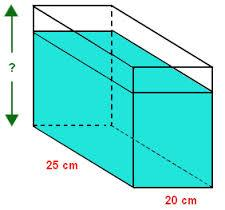Did you find an error or inaccuracy? Feel free to write us. Thank you!Tips to related online calculators
Do you have a system of equations and looking for calculator system of linear equations?
Tip: Our volume units converter will help you with the conversion of volume units.
Tip: Our Density units converter will help you with the conversion of density units.

## Related math problems and questions:

• Cork and swimmingIf a person weighs 80 kg, how many kilograms of cork must take a swimming belt to float on water? The density of the human body is 1050kg/m3 and cork 300kg/m3. (Instructions: Let the human body and cork on a mixture with a density of 1000kg/m3. When you m
• Barrel 3Barrel with water weights 118 kg. When we get off 75% of water, it weights 35 kg. How many kg has an empty barrel?
• Container with water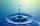The weight of a container with water is 2.48 kg. If the cast 75% water, the container with water has a weight of 0.98 kg. Determine the weight of the empty container. How much water was originally in the container?
• Water wessel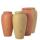The vessel containing water has a weight 19 kg. After pouring half the water its weigth 11 kg. What is the weight of the empty vessel?
• RingThe ring made from gold and copper alloy has a weight of 14.5 g and a volume of 1.03 cm3. How much gold and how much copper it contains? The metal densities are Au 19.3 g/cm³ and Cu 8.94 kg·dm-3
• Water containerContainer with water weighs 1.48 kg. When we cast 75% of water container of water weight 0.73 kg. How heavy is an empty container?
• Barrel 4Barrel of water weighs 63 kg. After off 75% water, the weight of the barrel with water is 21 kg. How many kg weigh empty barrel and how many kgs water in it?
• Cuboid 5Calculate the mass of the cuboid with dimensions of 12 cm; 0.8 dm and 100 mm made from spruce wood (density = 550 kg/m3).
• Hollow sphereThe steel hollow sphere floats on the water plunged into half its volume. Determine the outer radius of the sphere and wall thickness, if you know that the weight of the sphere is 0.5 kg and the density of steel is 7850 kg/m3
• BucketThe bucket half filled with water weighs 5.55 kg, the full bucket weighs 9.85 kg. How much does the bucket weigh?
• CuZn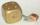Brass is an alloy of copper and zinc. The 10 centimeter brass cube has a weight of 8.6 kg. Copper density is 8930 kg/m3, the zinc density is 7130 kg/m3. Calculate how many kg of copper and zinc a cube contains.
• Empty canisterThe canister is filled with oil weight 17 kg. If filled only half weight 9 kg. What weight does my empty canister have?
• Watering can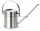A watering can full of water weighs 11 kg, the weight of water is 10 kg greater than the weight of an empty watering can, how much is the weight of the watering can?
• HomeworkMother and Kate together weigh 24.5 kg more than the father. Father weighs 7.6 kg more than mother. Father weight 44.8 kg more than Kate. Determine weights of father mother and Kate.
• The water barrel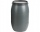The water barrel weighs 122 kg. If we pour 75% of the water out of it, it will weigh 35 kg. What is the weight of the barrel?
• Above water surface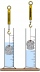If we remove the stone from the water, we apply a force of 120N. How much force will we have to exert if we move the stone above the water surface? The density of the stone is 5000 kg/m ^ 3.
• Center of gravityThe mass points are distributed in space as follows - specify by coordinates and weight. Find the center of gravity of the mass points system: A1 [1; -20; 3] m1 = 46 kg A2 [-20; 2; 9] m2 = 81 kg A3 [9